xPressure gradient forceEncyclopedia
The pressure gradient force is not actually a 'force' but the acceleration of air due to pressure difference (a force per unit mass). It is usually responsible for accelerating a parcel of air from a high atmospheric pressure
Atmospheric pressure
Atmospheric pressure is the force per unit area exerted into a surface by the weight of air above that surface in the atmosphere of Earth . In most circumstances atmospheric pressure is closely approximated by the hydrostatic pressure caused by the weight of air above the measurement point...

region to a low pressure region, resulting in wind
Wind
Wind is the flow of gases on a large scale. On Earth, wind consists of the bulk movement of air. In outer space, solar wind is the movement of gases or charged particles from the sun through space, while planetary wind is the outgassing of light chemical elements from a planet's atmosphere into space...

. In meteorology, pressure gradient force refers to the horizontal movement of air according to the equation: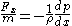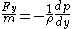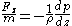The term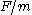is equal to the acceleration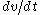because this is an expression of Newton's law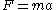.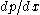is the component of the pressure gradient along the x-axis.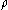is the mass density and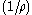shows that as the mass density increases, the acceleration due to the pressure gradient becomes smaller.

The pressure gradient force acts at right angles to isobars in the direction from high to low pressure. The greater the pressure difference over a given horizontal distance, the greater the force and hence the stronger the wind.

The pressure gradient force, however, is not the only force that acts on a moving parcel of air — if it were, then low and high pressure regions would eventually disappear. Other forces acting on a horizontally moving parcel of air include; surface friction
Friction
Friction is the force resisting the relative motion of solid surfaces, fluid layers, and/or material elements sliding against each other. There are several types of friction:...

, coriolis force, centrifugal force. In large-scale atmospheric flows, the coriolis force generally balances the pressure gradient force, producing winds blowing largely along the isobars; however, near the surface the friction term is also important, generally giving a resulting net wind direction diagonal to the isobars (with a component blowing towards the low pressure center).
The source of this article is wikipedia, the free encyclopedia.  The text of this article is licensed under the GFDL.